Algebra

# Stretching Graphs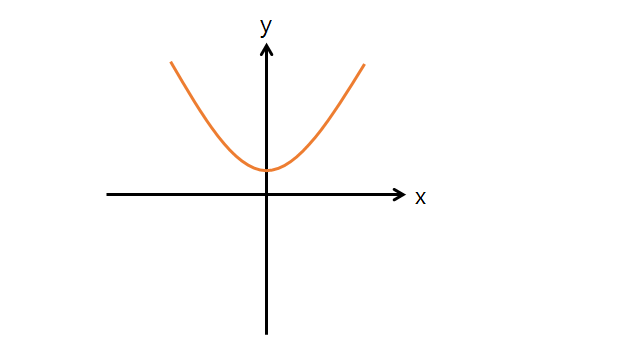Given the graph $y = 4x^2+9$, how do we obtain the graph of $y = 196x^2 +9?$

Note: The above graph is not drawn to scale.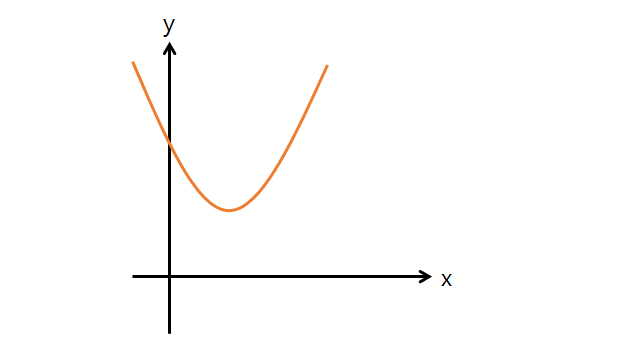What is the equation of the resulting graph after stretching $y=9(x-4)^2+8$ by a factor of $\ 5$ in the $y$-direction?

Note: The above graph is not drawn to scale.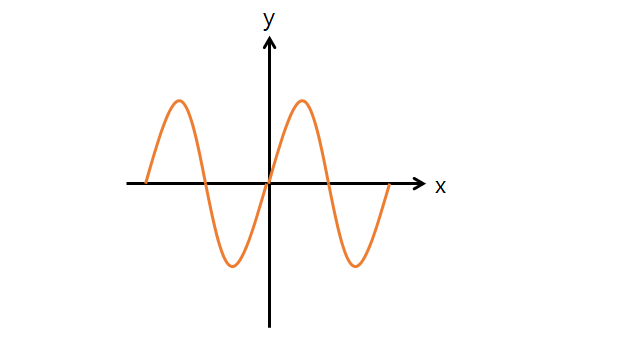What is the equation of the resulting graph after stretching $y=7\sin(8\pi x)$ by a factor of $\frac{1}{8}$ with respect to the $x$-axis?

Note: The above graph is not drawn to scale.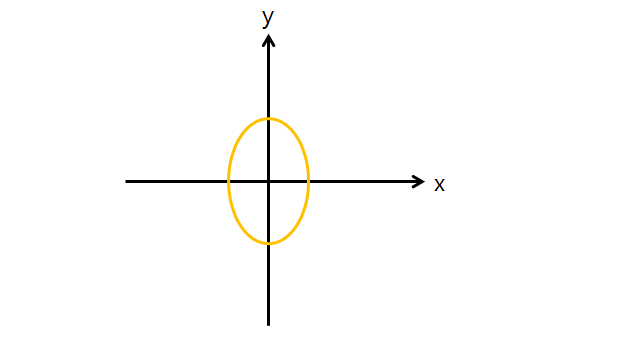If the graph of $49x^2+9y^2=25$ undergoes stretching by a factor of $a > 0$ with respect to the $x$-axis and stretching by a factor of $b >0$ with respect to the $y$-axis such that the resulting graph is a circle of radius $5$, what are $a$ and $b?$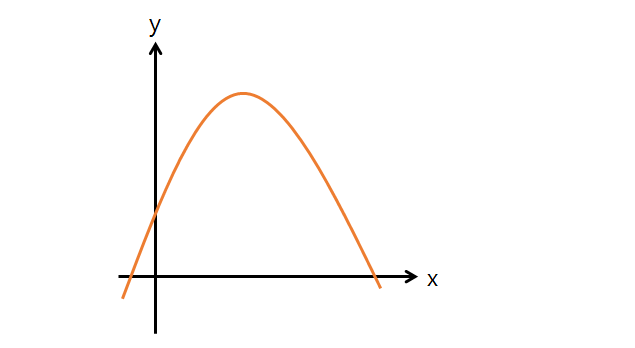What is the equation of the resulting graph after stretching $y=-8x^2+9x+6$ by a factor of $\frac{1}{6}$ horizontally and by a factor of $2$ vertically?

Note: The above graph is not drawn to scale.

×